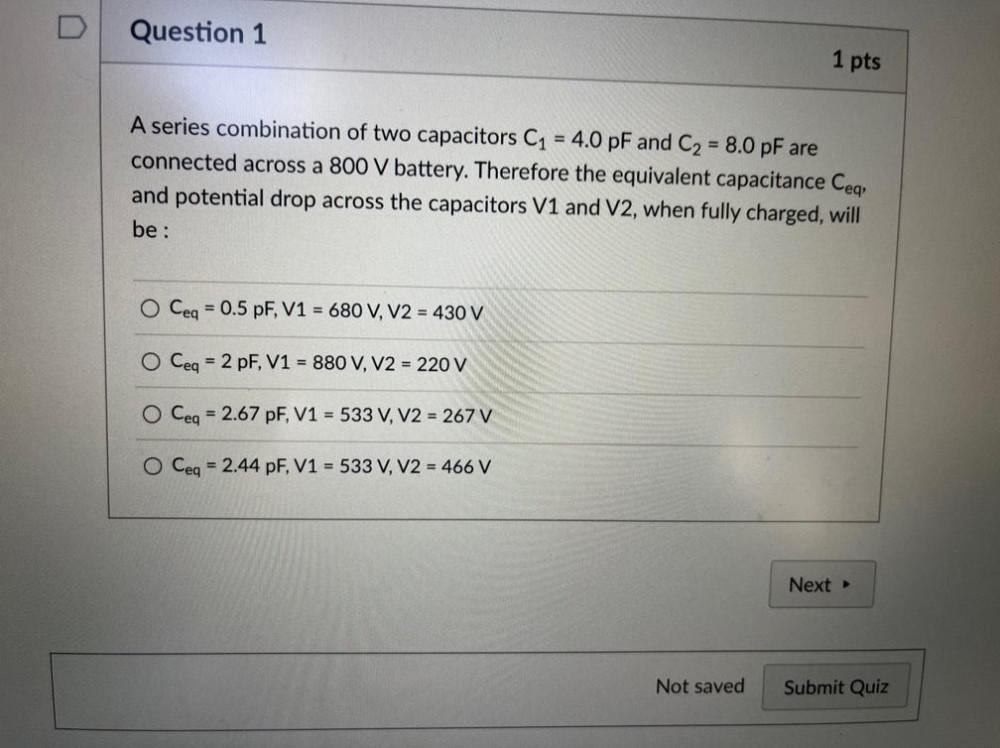Question:

# D Question 1 1 pts = A series combination of two capacitors C1 = 4.0 pF and C2 = 8.0 pF are connected across a 800 V battery. ThD Question 1 1 pts = A series combination of two capacitors C1 = 4.0 pF and C2 = 8.0 pF are connected across a 800 V battery. Therefore the equivalent capacitance Ceq, and potential drop across the capacitors V1 and V2, when fully charged, will be : O Ceq = 0.5 pF, V1 = 680 V, V2 = 430 V O Ceq = 2 pF, V1 = 880 V, V2 = 220 V O Ceq = 2.67 pF, V1 = 533 V, V2 = 267 V O Ceq = 2.44 pF, V1 = 533 V, V2 = 466 V Next Not saved Submit Quiz